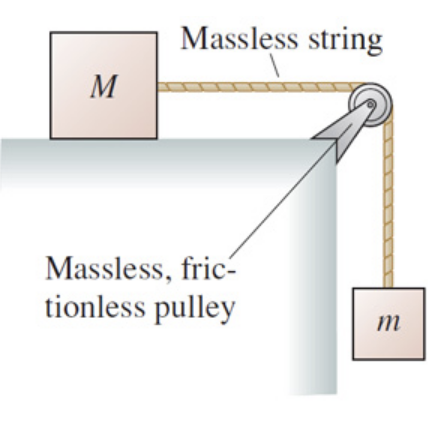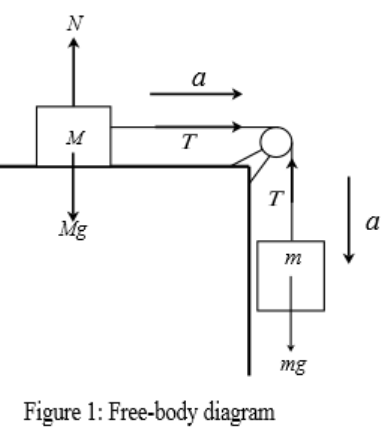In: Physics

# The block of mass M in the following figure slides on a frictionless surface. (Figure 1)The block of mass $$\mathrm{M}$$ in the following figure slides on a frictionless surface. (Figure 1)

Find an expression for the tension in the string.

Express your answer in terms of the variables $${m},{M}$$, and appropriate constants.

## Solutions

##### Expert Solution

Concepts and reason

The main concept required to solve this problem is Newton's second law of motion. First, draw a free body diagram describing the whole system. Then balance the forces acting in the horizontal and vertical directions and determine the expression for tension using Newton's second law of motion.

Fundamentals

Newton's second law of motion states that the net force acting on an object is directly proportional to the product of its mass and acceleration. According to Newton's second law, the net force acting on an object is, $$F=m a$$

Here, $$\mathrm{m}$$ is the mass of the object, and $$\mathrm{a}$$ is the acceleration of the object. The weight of an object is the force on an object due to gravity. Force acting on an object due to its weight is, $$F_{\mathrm{W}}=m g$$

Here, $$\mathrm{m}$$ is the mass of the object, and $$\mathrm{g}$$ is the acceleration due to gravity.

The following figure shows the free body diagram of two masses connected through a string over a pulley. The block of mass m is accelerating with acceleration in the downward direction, and the block of mass M is accelerating with the same acceleration towards the right. The tension in the string is T. N is the normal force acting on the block of mass M in the upward direction, and Mg is the force acting on the block due to gravity. The force on the block of mass m due to gravity is mg.As the two blocks are connected through a string, the two blocks accelerate with the same acceleration. The force on an object of mass $$\mathrm{m}$$ due to gravity is given as, $$F_{\mathrm{W}}=m g$$

Here, $$\mathrm{g}$$ is the acceleration due to gravity.

Refer to figure 1 and determine the net force acting on the block of mass $$\mathrm{M}$$ in the horizontal direction. $$F_{1}=T$$

Here, $$T$$ is the tension in the string. Substitute Ma for $$F_{1}$$ in equation $$F_{1}=T$$ and solve for $$a$$.

$$M a=T$$

$$a=\frac{T}{M}$$

Refer to figure 1 and determine the net force acting on the block of mass $$\mathrm{m}$$. $$F_{2}=m g-T$$

Here, $$\mathrm{g}$$ is the acceleration due to gravity, and $$\mathrm{T}$$ is the tension in the string. Substitute ma for $$F_{2}$$ in equation $$F_{2}=m g-T$$. $$m a=m g-T$$

Substitute $$\frac{T}{M}$$ for $$\mathrm{a}$$ in equation $$m a=m g-T$$ and solve for $$\mathrm{T}$$.

$$m\left(\frac{T}{M}\right)=m g-T$$

$$T\left(\frac{m}{M}+1\right)=m g$$

$$T=\frac{m M g}{m+M}$$

According to Newton's second law, the net force acting on an object is equal to the product of its mass and acceleration. The net force the block of mass $$\mathrm{m}$$ is. $$F_{2}=m a$$

Here, a is the acceleration of the block. The net force acting on the block of mass $$\mathrm{M}$$ is, $$F_{2}=M a$$

The forces ( $$\mathrm{N}$$ and $$\mathrm{Mg}$$ ) acting on the block of mass $$\mathrm{M}$$ are balanced out. Hence, the net force acting on the block of mass $$\mathrm{M}$$ in the vertical direction is zero, and the block accelerates towards the right. The tension on block $$\mathrm{M}$$ also acts towards the right. Thus, the net force acting on the block is, $$F_{1}=T$$

The tension on block $$\mathrm{m}$$ is in the upward direction. The weight of block $$\mathrm{m}$$ acts in the downward direction, and the block also accelerates in the downward direction. Thus, the net force acting on the block is, $$F_{2}=m g-T$$

The expression for the tension in the string is $$\frac{m M g}{m+M}$$.